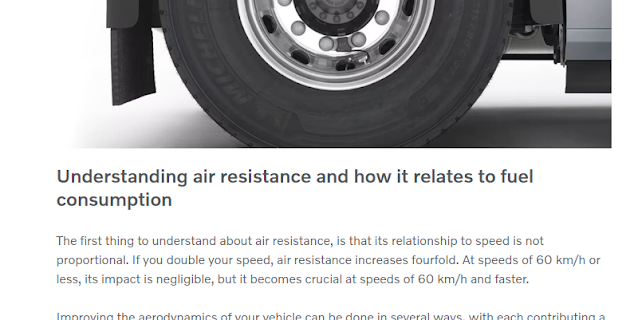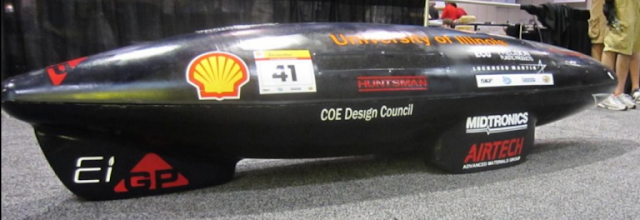### Common Misconceptions in Aerodynamics: Part 9

Aerodynamics Matter Regardless How Fast a Car Goes

The claim: Drag only matters if you go above a certain speed. 45 mph/50 mph/55 mph/60 mph—take your pick, as the critical speed depends entirely on the person reporting this myth.

The reality: People who repeat this claim are telling only part of the story—as is true of many, if not all, of these misconceptions. To see why, we need to go back (again) to some fundamentals of fluids in motion.

If you’ve ever taken a college physics class, you might remember something called the “Bernoulli equation.” This equation describes the relationship between internal energy, potential energy, and kinetic energy in a fluid—not just a fluid in motion, but any (incompressible) fluid (e.g. the “hydrostatic equation” is the Bernoulli equation simplified for a static fluid). By definition, the specific volume, v, of an incompressible fluid must remain constant, allowing us to divide out volume, V, and convert the energy terms into pressures. Because energy is conserved (that is, it is neither created nor destroyed), the sum of internal energy + potential energy + kinetic energy must be constant in a system if no energy flows cross the system boundary and if mass flow in = mass flow out (that is, the system is in steady state). In mathematical terms,
Assuming no energy is added or taken away from the system (and assuming an incompressible fluid, etc.), this relationship should hold true.

You might recognize one of these terms from the equation for aerodynamic drag force (which is one component of total aerodynamic force, acting parallel to the car’s centerline):
One-half of the product of density and the square of velocity is something we call “dynamic pressure,” and it is, as its form suggests, simply the kinetic energy of an incompressible flow with volume divided out (recall that the kinetic energy of a solid is equal to one half of the product of its mass and the square of its velocity. Dividing mass m by volume V gives density ρ; the inverse of this, V/m, is specific volume, v). In ground vehicle aerodynamics, gravity can (usually) be ignored since its effect on airflows is negligible when considering the flow around a car. This leaves a modified form of Bernoulli’s equation,
As you might guess, this is where the negative relationship between pressure and velocity is described: as one goes up, the other must go down to satisfy the equation. Rewriting the equation makes this clearer:
(Fun fact: you can also derive this relationship between velocity and pressure from an energy model of an incompressible fluid moving through a converging duct [nozzle] or diverging duct [diffuser], as well as a more complicated method involving vector math and linear algebra).

Consequently, measuring panel pressures on a moving car tells you something about the airflow at that location, namely whether it is faster (negative gauge pressure) or slower (positive gauge pressure) than the car’s speed.

Now let’s look at the kinetic energy term, or dynamic pressure, specifically. This is proportional to the square of velocity, and since aerodynamic force is directly related to dynamic pressure, drag is also proportional to the square of the flow velocity. This means that as a car’s speed increases, the aerodynamic drag it must overcome goes up dramatically faster. A car traveling at 60 mph must overcome 4 times as much aerodynamic drag as it did at 30 mph, even though its speed has only doubled.

The other force fighting a car in motion is what we call “rolling” or “mechanical” drag, which includes things like the friction and inertia of rotating engine and driveline components such as axles, wheels, and tires. At low speeds, aerodynamic drag is a much smaller proportion of the total resistance acting against a car than mechanical drag, and depending on the car (and especially how much mass it has, how big it is, and how it is shaped), they can be equal anywhere between, roughly, 40-60 mph. If that seems like a wide range, well—there are a lot of different cars out there, from tiny hatchbacks to hulking pickup trucks to Class 8 tractor-trailers, with widely varying drag characteristics. So, the first problem with the assertion that aerodynamic drag “doesn’t matter” below a certain speed is that the speed where aerodynamic drag becomes predominant will vary depending on the car. A blanket statement pinpointing one exact speed for every car or truck is simply incorrect.

The second problem is aerodynamic drag varies continuously. Say we’re looking at a car where aero drag equals mechanical drag at 60 mph. Well, what about at 59 mph? Aero drag is still almost equal to mechanical drag at that speed in our hypothetical car. How about 58 mph? 57 mph? 56 mph? Would reducing the drag coefficient of the car still “matter” at 55 mph even though at that speed aerodynamic force is only around 85% as large as mechanical resistance?Drag doesn’t suddenly matter at 60 kph—it isn’t an on/off switch. (Also, this should read "its relationship to speed is not directly proportional"—since it is proportional to speed squared!).
I would argue it does; resistive force is resistive force. Cut the car’s speed in half, to 30 mph, and now you might be able to make a case for ignoring improvements to its aerodynamic performance since that aero drag is now only 25% as large as mechanical drag in our hypothetical example (at the same time, what car only gets driven at 30 mph? Okay, aside from Supermileage bullet cars:Even though they race at low speeds, these cars are optimized for low aerodynamic drag. Since they are also very lightweight, aero drag might be as much or more than mechanical drag, even at low speed. (Image credit: https://ecoillini.mechse.illinois.edu/).

...and bicycles. The effects of aerodynamic drag--especially how it changes with body position and headwinds--can be easily felt on a bicycle, and measured. For example, my best 40 km time trial on this bike:

...was 62 minutes. However, on this bike:

...that improved to 56 minutes, more than 4 kph faster).

But there is no discrete point at which aero drag “matters” and below which it doesn’t, only a speed above which aero drag predominates and below which it doesn’t but may still be substantial. And keep in mind, that critical speed is different (and usually unknown without sophisticated measuring equipment) for every car model.